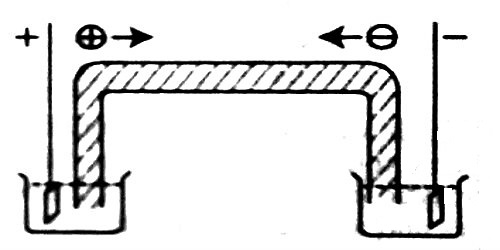Chemistry

# Absolute Velocity of Ions: Ionic MobilityAbsolute Velocity of Ions: Ionic Mobility

The difference in the ion conductances may be traced to the difference in the speeds with which the ions move under a given potential gradient. A direct measurement of the speeds with which ions move, i.e., their absolute velocities were first made by Oliver Lodge. A simple apparatus of the type as shown in Figure was used. In the tube was placed a gel into which was incorporated some indicating material. For example, in measuring the speed of hydrogen ion the anode and the cathode vessels are filled with dilute sulphuric acid and sodium sulphate. The tube is tilled with a gel of agar-agar to which phenolphthalein and a trace amount of alkali was incorporated so as to give a pink color to the gel. On passing electric current through the electrodes the hydrogen ion moves towards the cathode. The rate of movement is followed by nouns the rate of disappearance of the color in the gel due to neutralization of alkali by the hydrogen ions.Figure: Apparatus used by Lodge to measure the absolute velocity of ions

The success of the method depends on producing sharp boundaries and observing the movements of the boundaries accurately. However, the speeds will depend on the potential gradient across the electrodes. In order to compare the speeds of different ions a term ionic mobility has been introduced. The ionic mobility is defined as-

“The velocity of an ion in cm per second when it moves under unit potential gradient” i.e., when the potential between two electrodes placed 1 cm apart is 1 volt.”

The ionic mobility, u, is related to ion conductance λ by the expressions:

λa ∞ ua or, λa = K ua…. … (1)

and, λc ∞ uc or, λc = K uc … …. (2)

In equations (1) and (2), λa and λc are the ion conductance of anion and cation respectively and k is a constant of proportionality. The value of the constant k has been shown to be equal to one Faraday.

If we consider the ion conductance at infinite dilutions it can be shown that –

λ0a + λ0c = k (ua + uc)

These relations enable one to calculate the ionic mobility from the values of ion conductance.